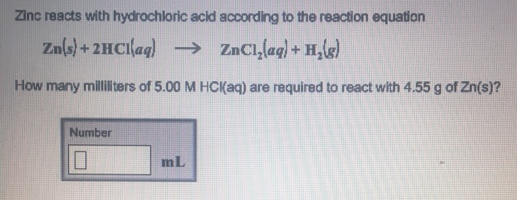# Problem: Zinc reacts with hydrochloric acid according to the reaction equation.Zn(s) + 2HCl(aq) → ZnCl2(aq) + H2(g)How many milliliters if 5.00 M HCl(aq) are required to react with 4.55 g of Zn(s)?

###### FREE Expert Solution
97% (355 ratings)###### Problem Details

Zinc reacts with hydrochloric acid according to the reaction equation.

Zn(s) + 2HCl(aq) → ZnCl2(aq) + H2(g)

How many milliliters if 5.00 M HCl(aq) are required to react with 4.55 g of Zn(s)?